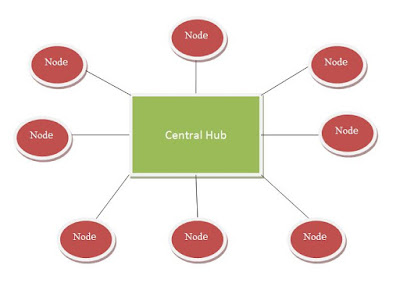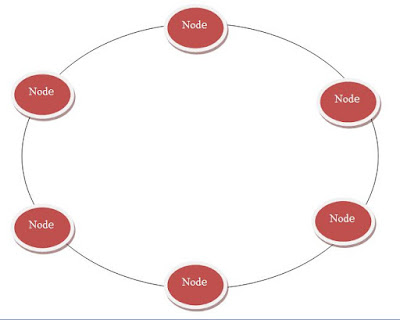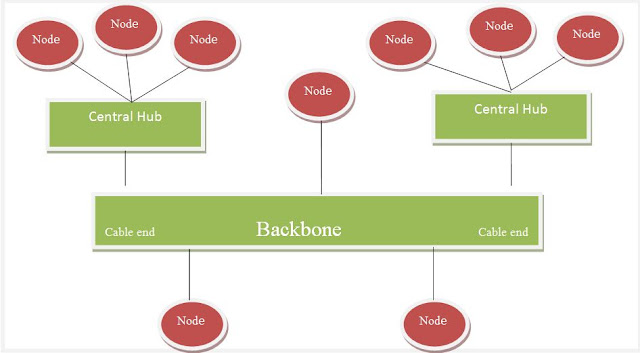## Network Topologies

### What is a network topology?

Networking topology is the outline of how to arrange interlinked devices in a network. Basically, it’s the graphical pattern in which the devices are arranged and rearranged in order to maintain the data traffic over the network. Network Topologies can be physical or may be logical (means has to be related to the signal).

The interlinked devices (are mainly called nodes) are the one's which are arranged in several ways, but the most important of the network topologies are,

1.   Mesh Topology
2.   Star Topology
3.   Bus Topology
4.   Ring Topology
5.   Hybrid Topology

### Mesh Topology

In a mesh topology, every node has a dedicated point-to-point link with every other node. The term dedicated means that the link carries traffic between two nodes. First we consider that each node must be connected to every other node. Now if there are ‘n’ no of nodes connected in a mesh topological network, then Node 1 must be connected to (n-1) nodes, Node 2 will also be connected to (n-1) nodes and then finally Node n should be connected to (n-1) nodes. See the figure below for better understanding.Network topology

The figure drawn above is the example of fully connected mesh network. Now, looking at the figure there are 4 nodes and each has n-1 no of links i.e. 4-1=3, and total of 6 links.

So, what is the total no of links if ‘n’ no of nodes are there?

Ans.
n (n-1)/2
= 4 (4-1)/2   [Taking n=4 nodes from the figure]
= (4x3)/2
= 6

### Star Topology

In a star topology, each node has a dedicated point-to-point link only with a fundamental controller (known as Hub). Here, no nodes are directly connected with each other. Unlike mesh network, a star network does not permit direct traffic between the nodes. But this controller acts as an organizer. One node must send the data to the controller and the controller conveys the data to the other nodes. Looking at the figure below, you will get the idea why it’s called the star topology.Network topology

### Bus Topology

Bus topology is based on multipoint connection, which has more than two specific nodes that shares a single link. This single cable link is also called backbone, which links to all devices in a network. See the figure for better understanding.Network Topology

### Ring Topology

A ring topology is easy to install and reorganize. In this topology, each node is dedicatedly point-to-point linked with only its immediate neighboring nodes. A signal is conceded in the ring in one direction until it arrives to its destination.Network topology

### Hybrid Topology

Hybrid topology is the pattern which can be composed of two or more different types of networking topologies. Like an example, we can comprise star topology with bus topology. See the figure below to understand it.Network topology

### Study Sessions

Q1. Explain the details of Ring topology.

Q2. Explain Star topology. What kind of hub is used in Star Topology?

Q3: Explain for 'n' number of devices, what can be the number of links or connections required for mesh, ring, and star topology?

PLEASE USE THE COMMENT SECTION FOR ANY DOUBTS AND QUERIES.